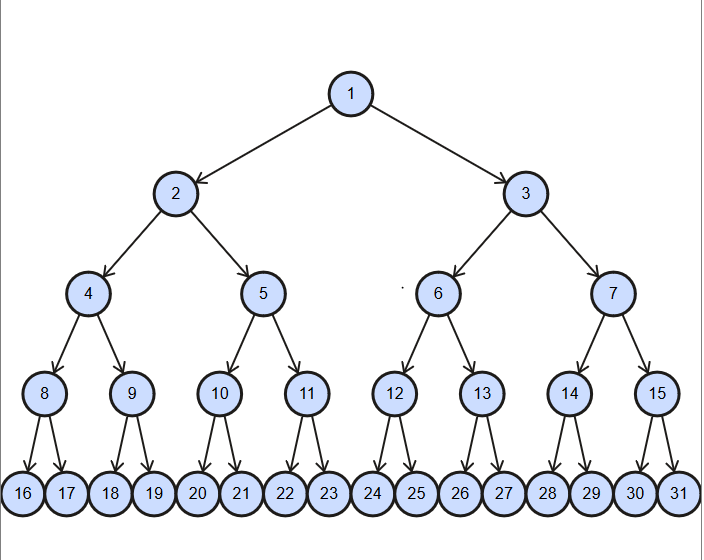# Consider a state space where the start state is number 1 and the successor function for state n returns two states, numbers 2n and 2n + 1.a) Draw the portion of the state space for states 1 to 31.b) Suppose the goal state is 12. List the order in which nodes will be visited for depth first search if we start with the left side of the tree, depth first search if we start with the right side of the tree, and breadth first search.c) Calculate the total time in terms of number of nodes each algorithm will take to find the goal state 12.

Question
135 views

Consider a state space where the start state is number 1 and the successor function for state n returns two states, numbers 2n and 2n + 1.

a) Draw the portion of the state space for states 1 to 31.

b) Suppose the goal state is 12. List the order in which nodes will be visited for depth first search if we start with the left side of the tree, depth first search if we start with the right side of the tree, and breadth first search.

c) Calculate the total time in terms of number of nodes each algorithm will take to find the goal state 12.

check_circle

Step 1

a) Since the state space starts from state number 1 and the successor function for state n returns two states, numbers 2n and 2n + 1, the portion of the state space for states 1 to 31 will be following:Step 2

b) The start state is 1 and the goal state is 12.

The order in which nodes will be visited for depth first search if we start with the left side of the tree:

1-->2-->4-->8-->16-->17-->9-->18-->19-->5-->10-->20-->21-->11-->22-->23-->3-->6-->12.

The order in which nodes will be visited for depth ...

### Want to see the full answer?

See Solution

#### Want to see this answer and more?

Solutions are written by subject experts who are available 24/7. Questions are typically answered within 1 hour.*

See Solution
*Response times may vary by subject and question.
Tagged in

### Computer Science